Study Guide

# Polynomials - Use Polynomial Identities to Solve Problems

## Use Polynomial Identities to Solve Problems

Pack up your gear and move out. We're trekking further up Polynomial Mountain. Along the way, we'll do more factoring, now with perfect square trinomials and the difference of two squares. Keep on the lookout for sums and differences of cubes, too. We'll need a few of those. Once we've gathered these all up, we can search on for the great Binomial Theorem at the summit.

### Binomials, Trinomials, and Squares, Oh My

Keep your eyes on the trail up Polynomial Mountain. Just ahead we can see some binomials that have been squared.

Our polynomial tour guide tells us that one special case of factoring involves squared binomials. Our guide leans in close and whispers another little trick in our ears (because we just don't have enough of those):

"The product of a squared binomial is a perfect square trinomial."

She then passes us a note with the following on it:

(a + b)2 = (a + b)(+ b) = a2 + 2ab + b2

(ab)2 = (b)(ab) = a2 – 2ab + b2

She then nods, factors herself into a squared binomial, and rolls down the mountain. Well, that was weird.

Look carefully at that note, mathronaut. Notice that the first and last terms of the trinomial are both perfect squares, and that the trinomial's middle term is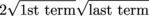. Also note that the sign before the middle term matches the sign in the binomial.

It's perfect. It has squares. It's a trinomial. Hence the name.

We could figure all of this out just by multiplying the squared binomial out. The important part is that this relationship works in both directions; the reverse is also true:

A perfect square trinomial factors to a binomial squared. Check it out:

a2 + 2ab + b2 = (a + b)2

a2 – 2ab + b2 = (ab)2

How about we put this to work?

### Sample Problem

Factor x2 – 2x + 1.

Okay, mathronaut. Is it a perfect square trinomial?

Well, the first and last term are perfect squares.

Does the middle term equal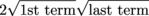?

Yep. The square root of x2 is just x, the square root of 1 is 1, and 2 times all that is 2(x)(1) = 2x. This factors out to a squared binomial. How convenient.

Just take the square root of the first term and the square root of the last term, throw a "–" sign between them, and square the whole shebang.

x2 – 2x + 1 = (x – 1)2

Let's keep this train moving.

### Sample Problem

Factor 25x2 + 20x + 4.

We can see that the first and last terms are perfect squares, of 5x and 2. Looking good so far.

How about the middle term? Does 20x = 2(5x)(2)? Hyup, it sure does.

This is a perfect square trinomial, which factors to a squared binomial.

What's a mathronaut to do? Panic? No, we'll take the square roots of the first and last terms, throw in a "+" sign between them, and then square the whole thing.

25x2 + 20x + 4 = (5x + 2)2

Okay, just one more to go before we move on.

### Sample Problem

Factor 9x4 – 6x2 + 1.

Well, 9x4 is the square of 3x2, and 1 is the square of…1. They seem pretty perfect to us, so that checks out.

Does 6x2 = 2(3x2)(1)? Why yes indeed. The middle term matches our expectations. This is a perfect square trinomial. We know how it factors from here.

9x4 – 6x2 + 1 = (3x2 – 1)2

Great, we've done it, and all without running into any lions, tigers, or bears. Now we can move on.

### Domestic Square Dispute, Or, The Difference Between Two Squares

Next, look to the left of the trail up Polynomial Mountain. We can see Captain Kirk climbing the mountain—why is he climbing the mountain?

Next to him, we see another special case of polynomial factoring: the difference of squares.

Our polynomial friends can barely contain themselves. They have another little trick to show us:

(a + b)(a b) = a2b2

(ab)(a + b) = a2b2

In words, a more math-y person might say, "The product of a sum and a difference is a difference of two squares." A less math-y person might say, "Golly, that's a lot of letters."

Just like the squared binomials, this works both ways.

a2b2 = (a + b)(ab)

### Sample Problem

Factor 9x2 – 4.

We definitely have a difference here; that's subtraction to most folks. 3x squares to 9x2, and 2 squares to 4, and you square to you2. Now there are two of you, so you can enjoy Shmoop twice as much.

We can now take the square root terms and multiply them together as a sum and a difference.

9x2 – 4 = (3x – 2)(3x + 2)

Done. Now let's trek on a little further and apply this concept to solving equations.

### Sample Problem

Solve 25x2 – 36 = 0 for x.

Our square roots terms are 5x and 6, so we plug those into our difference machine.

(5x + 6)(5x – 6) = 0

From here, it's business as usual for finding the roots for the equation.

5x + 6 = 0 and 5x – 6 = 0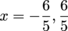Our business is concluded, so we'll be moving on before Kirk starts asking where all these zeros came from.

• ### Sum and Difference of Cubes

The air is getting thinner, so we must be near the peak of Polynomial Mountain. There are an unusual number of cubed terms around here. Two of them are getting rather close to each other. Here's the sum of two cubes.

a3 + b3 = (a + b)(a2ab + b2)

Whoa, they just split into a binomial multiplied by a trinomial. That must be a hit at polynomial parties.

Try and put them back together by multiplying things out:

(a + b)(a2ab + b2)

= a(a2ab + b2) + b(a2ab + b2)

= (a3a2b + ab2) + (a2bab2 + b3)

= a3 + b3

There we go, good as new. We noticed that for the sum of the cubes, the binomial has some addition in it, while the trinomial has a negative middle term. The difference of two cubes looks a little different.

a3b3 = (ab)(a2 + ab + b2)

The binomial shares their negative sign, while the trinomial has a totally positive outlook.

Let's keep a positive outlook ourselves while we work a...

### Sample Problem

Factor x3 + 27.

Shmooper, can we see that both terms are cubes? Aye-aye, we can see it.

We can think of it as:

(x)3 + (3)3

We saw before that this will factor to a binomial with positive terms, and a trinomial with a negative term in the middle. In this case, a = x and b = 3.

(x + 3)(x2 – 3x + 9)

We can multiply this out to check our work.

(x3 – 3x2 + 9x) + (3x2 – 9x + 27)

= x3 + 27

Checkmate, sample problem.

### Sample Problem

Factor a6b3.

Don't be fooled, mathronaut. This polynomial just came from a costume party at the top of the mountain. It really is a difference of two cubes.

(a2)3 – (b)3

Exponents raised to another exponent multiply together, remember? This wasn't that good of a costume if we can see through it so easily. Sorry, but we tell it like it is.

Now, about factoring this out. Our cubes are being subtracted, so the binomial is too, while the trinomial is full of pluses.

(a2b)[(a2)2 + a2b + b2]

Simplifying, we get:

(a2b)(a4 + a2b + b2)

We suggest that next year, little polynomial, you go as Batman. Actually, we suggest that every year.

### Sample Problem

Factor 64(x + y)3 + 27y3.

We have another costumed crusader here. This time, they've disguised their variables to look more complicated than they actually are. It's still a sum of two cubes, though.

Rewrite as:

[4(x + y)]3 + (3y)3

That makes it more obvious. Our terms are 4(x + y) and 3y. We can work with this; it's just going to require a full corset of parentheses, brackets, and braces.

[4(x + y) + 3y]{[4(x + y)]2 – 4(x + y)(3y) + (3y)2}

Simplifying this sounds like a good idea.

= (4x + 4y + 3y)[16(x2 + 2xy + y2) + (-12xy – 12y2) + 9y2]

= (4x + 7y)(16x2 + 32xy + 16y2 – 12xy – 3y2)

= (4x + 7y)(16x2 + 20xy + 13y2)

That's simple enough, we think. We wanted to factor it, after all.

Something interesting to note from these last few problems. Even though we had a trinomial come out of all of them, none of those trinomials could be factored. This will, in fact, almost always be the case.

That trinomial is a fast-talking rookie who prefers to work alone. He's been partnered with binomial, a long-time veteran of the force who plays by the rules and is only a few days away from retirement. Together, they fight crime.

• ### Pascal's Triangle and The Binomial Theorem

Good job, mathronaut! We're nearly to the pinnacle of Polynomial Mountain. At the top we'll plant our Shmoop flag high, snap a few photos to make the folks back home jealous, and find Pascal's Triangle and the Binomial Theorem.

These are both ways to quickly multiply out a binomial that's being raised by an exponent. Like, say:

(a + b)0 = 1

(a + b)1 = a + b

(a + b)2 = a2 + 2ab + b2

(a + b)3 = (a + b)(a + b)2 = (a + b)(a2 + 2ab + b2) = a3 + 3a2b + 3ab2 + b3

And so on. We could keep going all day, but eventually the exponents would get so big that it would take us all day to simplify them. We need a better way, and luckily a 17th-century French mathematician has already found one.

Blaise Pascal was an interesting dude. He studied physics, philosophy, religion, and mathematics—with maybe just a little help from alien polynomials from a certain planet. He found a numerical pattern, called Pascal's Triangle, for quickly expanding a binomial like the ones above.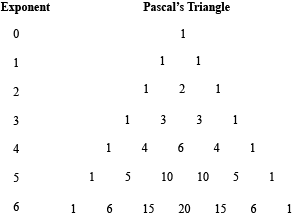Check it out. The first row is one 1. Then we have two 1s. After that, things get interesting. The outsides of the triangle are always 1, but the insides are different. To find the number on the next row, add the two numbers above it together. See, 2 = 1 + 1; for the row under that, we have 1 (outer edge), 3 (2 + 1 from above), 3 (ditto), and 1 (the other edge). Every row is built from the row above it.

Pascal's Triangle gives us the coefficients for an expanded binomial of the form (a + b)n, where n is the row of the triangle. The Binomial Theorem tells us we can use these coefficients to find the entire expanded binomial, with a couple extra tricks thrown in.

What about the variables and their exponents, though? Keep your pants on; the Binomial Theorem has us covered. At the left side of the triangle, we have anb0 = an. As we move to the right, we subtract 1 from a's exponent and add it to b's. It's like b keeps stealing exponents from a, one at a time, until he's got them all. That sneaky devil.

Pascal doesn't tell us about the sign of each term, though. Luckily, our polynomial friends have us covered. We're getting covered a lot here, aren't we?

Anyway, when a binomial has a "+" sign, such as (a + b)2, all of the terms of the expansion are positive.

When we expand a binomial with a "–" sign, such as (ab)5, the first term of the expansion is positive and the successive terms will alternate signs.

With all this help from Pascal and his good buddy the Binomial Theorem, we're ready to tackle a few problems.

### Sample Problem

Expand (xy)4.

Take a look at Pascal's triangle. From the fourth row, we know our coefficients will be 1, 4, 6, 4, and 1. That negative sign means that the first term of our expansion will be positive, and the following terms will alternate signs. The exponents will start at x4y0 and move to x3y1, etc.

(xy)4

= 1x4 – 4x3y + 6x2y2 – 4xy3 + 1y4

= x4 – 4x3y + 6x2y2 – 4xy3 + y4

Wasn't that much easier than trying to multiply the expression out? We probably wouldn't have even tried, no matter how much the polynomials complained to us.

### Sample Problem

Expand (m + 2n)5.

Have Pascal, will travel (all the way to the answer). Our coefficients are 1, 5, 10, 10, 5, 1. The 2n doesn't change anything; treat it like a single term.

1m5 + 5m4(2n) + 10m3(2n)2 + 10m2(2n)3 + 5m(2n)4 + 1(2n)5

= m5 + 10m4n + 40m3n2 + 80m2n3 + 80mn4 + 32n5

With Pascal on our side, we feel invincible. However, that isn't quite the case. We're still weak to bullets, being dipped in lava while a villain monologues at us, or a well-timed chocolate. We also have trouble dealing with very large exponents; for every row we add to Pascal's triangle, it takes longer and longer to find the next. We also can't afford to skip any rows, or the usher will escort us out.

Often times, when we have a binomial with a huge exponent, we only need one or a few terms. For those problems, we'll use a special formula. It allows us to find the coefficient of just one specific term without finding any of the others.

It looks pretty weird, but stay with us.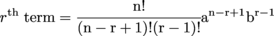Whew, that thing looks beastly. Just dreadful. It has fractions and exponents and factorials. We haven't even mentioned yet that the formula doesn't even tell you the sign of the term, either.

To find the rth term of a binomial expansion (a + b)n, plug the terms into the formula.

If the binomial has a "+" sign, then all terms found using this formula are positive.

If the binomial has a "–" sign, then the term is negative if r is even. If r is odd, then the term is positive.

Maybe it won't be so bad once we solve a problem or two with it. Don't judge a book-sized formula by its cover, eh?

### Sample Problem

Find the 12th term of (xy)14.

That binomial would take forever to multiply out, and we'd make hundreds of mistakes while doing it anyway. We're convinced already; give us the new formula, please.

Let's see: r = 12 and n = 14, while a = x and b = y. The 12th term is even, so our term will be negative.Remember, a factorial is a shortcut for writing a bunch of multiplication, like 3! = 3 × 2 × 1. That means we can cancel out a lot of that 14! with the 11! below it, since 14! = 14 × 13 × 12 × 11!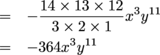Okay, we were skeptical at first, but this wasn’t actually too bad. As long as we know the formula, it works out.

### Sample Problem

Find the 7th term of (x + y)17.

This is it—the last problem before the summit of Polynomial Mountain. We've come a long way, so let's push just a little farther.

We have an r of 7 and an n of 17. The term will be positive because we've got a plus sign in our binomial, so all the terms are gonna be positive.Just think of how many terms we'd have to add together to get a number like 12,376 out of Pascal's Triangle.

With that out of the way, we've made it to the top. We've conquered Polynomial Mountain by learning the polynomials' ways, living among them, and befriending them in their alien home. Until next time, mathronauts!